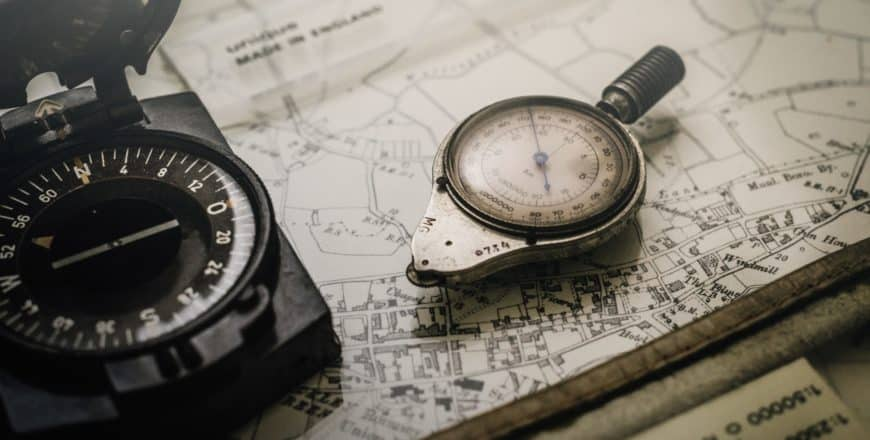# AS Maths VectorsThis AS Maths course (based originally on the Edexcel specification) teaches the fundamentals of the Vectors topics taught during the first year of A-Level Maths. These topics include:

• Magnitude and Direction of Vectors
• Unit Vectors
• Addition, Subtraction and Scalar Multiplication of Vectors
• Position Vectors
• Vectors and Trigonometry

There are 17 lessons which include notes, examples, and videos for the above topics followed by an assignments section with a quiz and a written assignment to sit and mark yourself.

We recommend that you allow 3 hours for completion of the AS Maths Vectors course.

Mark the bottom of each lesson ‘complete’ when you feel happy that you have learned the content. Happy Studying!

### Introduction

1
Introduction to AS Maths Vectors

This AS Maths course (based originally on the Edexcel specification) teaches the fundamentals of the Vectors topics taught during the first year of A-Level Maths. These topics include:

• Magnitude and Direction of Vectors
• Unit Vectors
• Addition, Subtraction and Scalar Multiplication of Vectors
• Position Vectors
• Vectors and Trigonometry

There are 17 lessons which include notes, examples, and videos for the above topics followed by an assignments section with a quiz and a written assignment to sit and mark yourself.

We recommend that you allow 3 hours for completion of the AS Maths Vectors course.

Mark the bottom of each lesson 'complete' when you feel happy that you have learned the content. Happy Studying!

### 2D Vectors

1
What are vectors?

A description of what vectors are

2
Magnitude and Direction of 2D Vectors
3
Scalar Multiplication of Vectors
4
Unit Vectors
5
2D Vectors Video 1

Finding the vector between two points and its magnitude.

6
2D Vectors Video 2

Using parallel lines to find the value of an unknown constant.

7
2D Vectors Video 3

Using magnitude to find the value of an unknown in a vector then using it to find a unit vector with calculations heavily based on surds.

### Vector Arithmetic

1
2
Vector Subtraction
3
Vector Arithmetic Video 1

Navigating along various known vectors to obtain unknown vectors and showing that three points are colinear.

4
Vector Arithmetic Video 2

Finding expressions for various vectors running along the perimeter of a trapezium.

5
Vector Arithmetic Video 3

A complicated vector arithmetic question involving the position vector of a point with unknown location.

### Vectors in Context

1
Position Vectors
2
Vectors and Trigonometry
3
Vectors in Context Video 1

Using points and position vectors to find a new position vector and using magnitude to find the value of an unknown constant.

### Assignments

1
AS Maths Vectors Quiz
5 questions
2
AS Maths Vectors Assignment